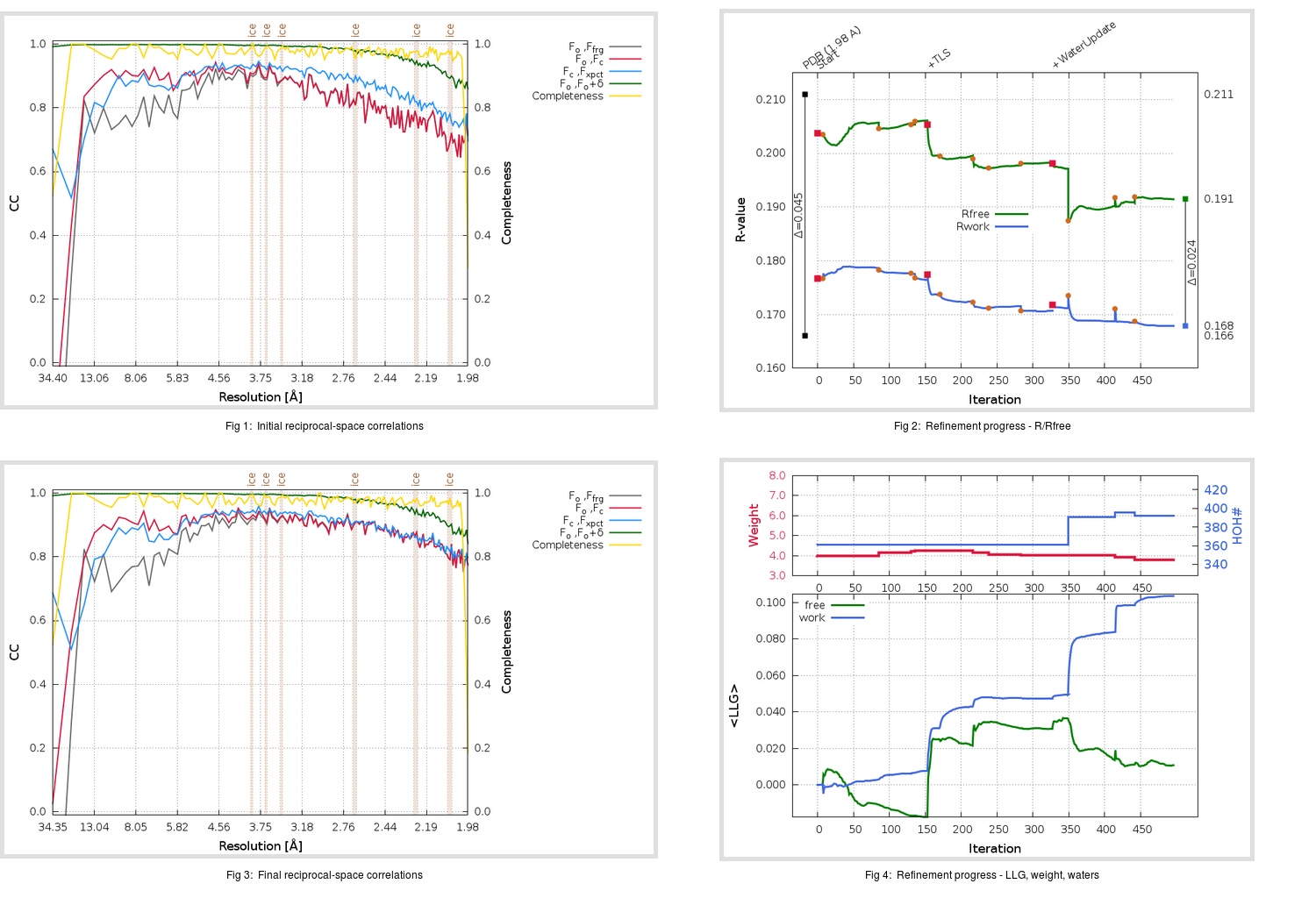Content:

```    Diffraction limits & principal axes of ellipsoid fitted to diffraction cut-off surface:
1.941         0.9583   0.0000   0.2858       a* + 0.021 c*
1.962         0.0000   1.0000   0.0000       b*
1.904        -0.2858   0.0000   0.9583      -0.502 a* + 0.865 c*
```

## Deposited

` `
 Date deposited Date data collection Resolution R, Rfree 20070719 20060826 1.98 0.1640 0.2110

Molprobity (CCP4 7.0 version) summary:

```Ramachandran outliers =   0.00 %
favored =  98.03 %
Rotamer outliers      =   1.86 %
C-beta deviations     =     1
Clashscore            =   2.49
RMS(bonds)            =   0.0160
RMS(angles)           =   1.63
MolProbity score      =   1.24
Resolution            =   1.98
R-work                =   0.1640
R-free                =   0.2110
```

```Number of waters      =   361

<B> (all atoms) =   33.06 ( sd =    8.94 ) for       2791 non-hydrogen atoms
<B>   (protein) =   31.62 ( sd =    7.95 ) for       2402 non-hydrogen atoms
<B>     (water) =   42.37 ( sd =    9.63 ) for        361 non-hydrogen atoms
<B>    (others) =   36.39 ( sd =    7.30 ) for         28 non-hydrogen atoms

B min/max       (all non-hydrogen atoms) =   17.46 /   69.68
B min/max   (protein non-hydrogen atoms) =   17.46 /   69.52
B min/max     (water non-hydrogen atoms) =   21.44 /   69.68
B min/max     (other non-hydrogen atoms) =   29.47 /   48.19
```

## BUSTER (re-)refinement

` `

Molprobity (CCP4 7.0 version) summary:

```Ramachandran outliers =   0.00 %
favored =  98.36 %
Rotamer outliers      =   1.49 %
C-beta deviations     =     0
Clashscore            =   1.24
RMS(bonds)            =   0.0116
RMS(angles)           =   1.59
MolProbity score      =   0.98
Resolution            =   1.98
R-work                =   0.1680
R-free                =   0.1915
```

```Number of waters      =   392

<B> (all atoms) =   34.64 ( sd =   10.03 ) for       2822 non-hydrogen atoms
<B>   (protein) =   32.72 ( sd =    8.20 ) for       2402 non-hydrogen atoms
<B>     (water) =   46.31 ( sd =   12.09 ) for        392 non-hydrogen atoms
<B>    (others) =   34.73 ( sd =    7.08 ) for         28 non-hydrogen atoms

B min/max       (all non-hydrogen atoms) =   19.83 /   91.20
B min/max   (protein non-hydrogen atoms) =   19.83 /   78.27
B min/max     (water non-hydrogen atoms) =   22.32 /   91.20
B min/max     (other non-hydrogen atoms) =   31.49 /   44.38
```

Refinement progression:Results:

` `
 File Remark 2V6N_aB_refine.01_03_refine.pdb.gz exact refinement commands are in header 2V6N_aB_refine.01_03_refine.mtz.gz including original deposited data and several re-refinement map coefficients 2V6N_aB_refine.01_03_BUSTER_model.cif.gz including any non-standard compound restraints 2V6N_aB_refine.01_03_BUSTER_refln.cif.gz